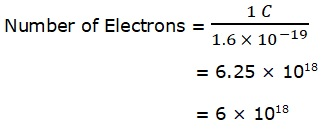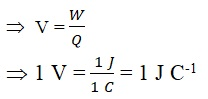Study Materials: ncert solutions

Our ncert solutions for Solutions 12. Electricity - Text-book Questions | Class 10 Science - Toppers Study is the best material for English Medium students cbse board and other state boards students.

Solutions ⇒ Class 10th ⇒ Science ⇒ 12. Electricity

# Solutions 12. Electricity - Text-book Questions | Class 10 Science - Toppers Study

Topper Study classes prepares ncert solutions on practical base problems and comes out with the best result that helps the students and teachers as well as tutors and so many ecademic coaching classes that they need in practical life. Our ncert solutions for Solutions 12. Electricity - Text-book Questions | Class 10 Science - Toppers Study is the best material for English Medium students cbse board and other state boards students.

## Solutions 12. Electricity - Text-book Questions | Class 10 Science - Toppers Study

CBSE board students who preparing for class 10 ncert solutions maths and Science solved exercise chapter 12. Electricity available and this helps in upcoming exams 2023-2024.

### You can Find Science solution Class 10 Chapter 12. Electricity

• All Chapter review quick revision notes for chapter 12. Electricity Class 10
• NCERT Solutions And Textual questions Answers Class 10 Science
• Extra NCERT Book questions Answers Class 10 Science
• Importatnt key points with additional Assignment and questions bank solved.

Chapter 12 Science class 10

### Text-book Questions class 10 Science Chapter 12. Electricity

• Solutions 12. Electricity - Text-book Questions | Class 10 Science - Toppers Study
• Class 10 Ncert Solutions
• Solution Chapter 12. Electricity Class 10
• Solutions Class 10
• Chapter 12. Electricity Text-book Questions Class 10

## Solutions 12. Electricity - Text-book Questions | Class 10 Science - Toppers Study

### Text-book Questions

Page no. 200

Q1. What does an electric circuit mean?

Ans: A continuous and closed path of an electric current is called an electric circuit. In which various electric components are arranged in series or parrallel.

Q2. Define the unit of current.

Ans: S.I unit of current is Ampere, which denoted by Letter 'A'. Ampere is defined as "When one coulomb of charge flows in one second it is callled one Ampere of current".

Q3. Calculate the number of electrons constituting one coulomb of charge.

Ans: One electron possesses a charge of 1.6 × 10-19 C,

Therefore, Charge on 1 electron = 1.6 × 10-19 C

Total Charge = 1 coulomb = 1C (given)

The number of elecrons = ?

Total Charge = number of electrons × Charge on 1 electron

1C = number of electrons × 1.6 × 10-19 CThe number of electrons constituting one coulomb of charge is 6 × 1018

Page no. 202

Q1. Name a device that helps to maintain a potential difference across a conductor.

Ans: Cell or Battery is a device that helps to maintain a potential difference across a conductor.

Q2. What is meant by saying that the potential difference between two points is 1 V?

Ans: If 1 J of work is required to move a charge of amount 1 C from one point to another then it is said that the potential difference between the two point is 1 V.Q3. How much energy is given to each coulomb of charge passing through a 6 V battery?

Ans: The energy given to each coulomb of charge is equal to the amount of work done to move it.

V = W/QPage no. 209

Q1. On what factors does the resistance of a conductor depend?

Q2. Will current flow more easily through a thick wire or a thin wire of the same material, when connected to the same source? Why?

Q3. Let the resistance of an electrical component remains constant while the potential difference across the two ends of the component decreases to half of its former value. What change will occur in the current through it?

Q4. Why are coils of electric toasters and electric irons made of an alloy rather than a pure metal?

Q5. Use the data in Table 12.2 to answer the following –

(a) Which among iron and mercury is a better conductor?

(b) Which material is the best conductor?

Page no. 213

Q1. Draw a schematic diagram of a circuit consisting of a battery of three cells of 2 V each, a 5 Ω resistor, an 8 Ω resistor, and a 12 Ω resistor, and a plug key, all connected in series.

Q2. Redraw the circuit of Question 1, putting in an ammeter to measure the current through the resistors and a voltmeter to measure the potential difference across the 12 Ω resistor. What would be the readings in the ammeter and the voltmeter?

Page no. 216

Q1. Judge the equivalent resistance when the following are connected in parallel – (a) 1 Ω and 106 Ω, (b) 1 Ω and 103 Ω, and 106 Ω.

Q2. An electric lamp of 100 Ω, a toaster of resistance 50 Ω, and a water filter of resistance 500 Ω are connected in parallel to a 220 V source. What is the resistance of an electric iron connected to the same source that takes as much current as all three appliances, and what is the current through it?

Q3. What are the advantages of connecting electrical devices in parallel with the battery instead of connecting them in series?

Q4. How can three resistors of resistances 2 Ω, 3 Ω, and 6 Ω be connected to give a total resistance of (a) 4 Ω, (b) 1 Ω?

Q5. What is (a) the highest, (b) the lowest total resistance that can be secured by combinations of four coils of resistance 4 Ω, 8 Ω, 12 Ω, 24 Ω?

Page no. 218

Q1. Why does the cord of an electric heater not glow while the heating element does?

Q2. Compute the heat generated while transferring 96000 coulomb of charge in one hour through a potential difference of 50 V.

Q3. An electric iron of resistance 20 Ω takes a current of 5 A. Calculate the heat developed in 30 s.

Page no. 220

Q1. What determines the rate at which energy is delivered by a current?

Q2. An electric motor takes 5 A from a 220 V line. Determine the power of the motor and the energy consumed in 2 h

Important Study materials for classes 06, 07, 08,09,10, 11 and 12. Like CBSE Notes, Notes for Science, Notes for maths, Notes for Social Science, Notes for Accountancy, Notes for Economics, Notes for political Science, Noes for History, Notes For Bussiness Study, Physical Educations, Sample Papers, Test Papers, Mock Test Papers, Support Materials and Books.Mathematics Class - 11th

NCERT Maths book for CBSE Students.

books

## Study Materials List:

##### Solutions ⇒ Class 10th ⇒ Science
1. Chemical Reactions and Equations
2. Acids, Bases and Salts
3. Metals and Non-metals
4. Carbon and its Compounds
5. Periodic Classification of Elements
6. Life Processes
7. Control and Coordination
8. How do Organisms Reproduce
9. Heredity and Evolution
10. Light-Reflection and Refraction
11. Human Eye and Colourful World
12. Electricity
13. Magnetic Effects of Electric Current
14. Sources of Energy
15. Our Environment
16. Management of Natural Resources

New Books